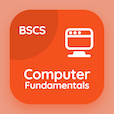SAT Online Courses

SAT Physics MCQs

SAT Physics MCQ PDF - Topics

# Work in Physics MCQ Quiz Online

Practice Work in Physics Multiple Choice Questions (MCQ), Work in Physics quiz answers PDF to learn sat physics online course for sat physics classes. Energy Mass and Power Multiple Choice Questions and Answers (MCQs), Work in Physics quiz questions for job placement test. "Work in Physics MCQ" PDF Book: power in physics, energy in physics, work in physics test prep for college entrance test.

"For 2 objects of the different masses moving at same speed, the object of greater mass has" MCQ PDF: work in physics with choices no kinetic energy, greater potential energy, smaller kinetic energy, and greater kinetic energy for job placement test. Learn work in physics quiz questions for merit scholarship test and certificate programs for online college admission.

## MCQs on Work in Physics Quiz

MCQ: For 2 objects of the different masses moving at same speed, the object of greater mass has

no kinetic energy
greater potential energy
smaller kinetic energy
greater kinetic energy

MCQ: A girl accidently dropped a stone from her building, the mass os the stone is 12 g and the height is 60 m. If the air resistance can be neglected and g = 10 N kg-1, what is the speed of the stone when it hits the ground?

5.6 J
7.2 J
3.9 J
5.8 J

MCQ: The SI unit of work is

Joule
kg-1
watt
Newton

MCQ: When a body of mass m moves at a speed v, its kinetic energy is defined as

Ek = mv2
Ek = 1/2 mv
Ek = (1/2)mv2
Ek = m/v2

MCQ: The product of the force and the distance moved by the object in the direction of the force is known as

effort
power
efficiency
work done

### More Topics from SAT Physics Course